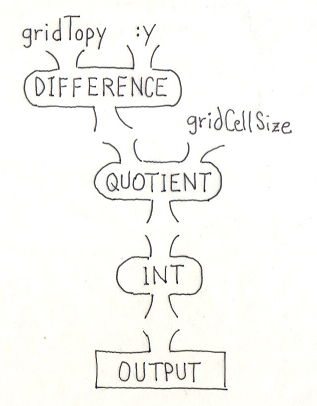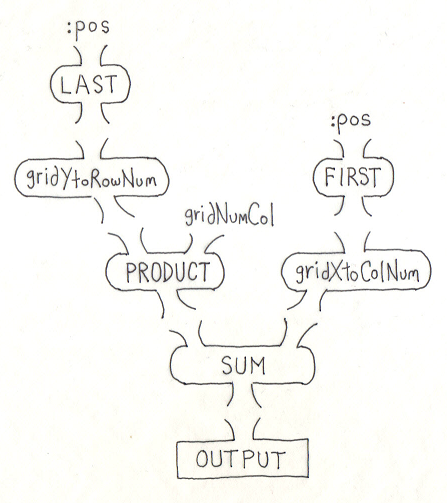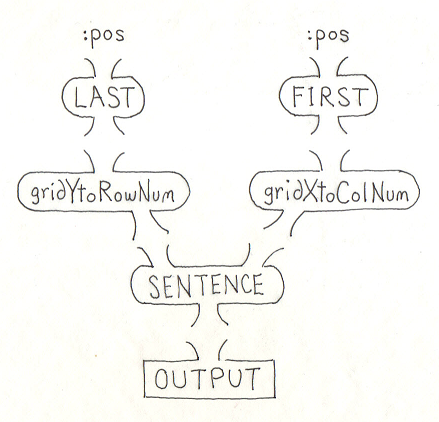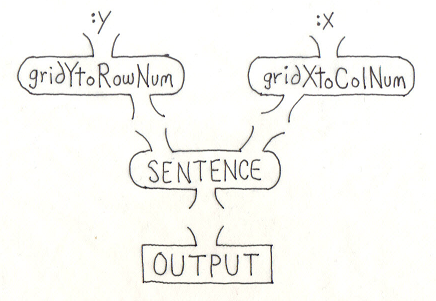BFOIT - Introduction to Computer Programming

# Practice Answers: New Grid Toolkit Procedures

### gridYtoRowNum1. DIFFERENCE outputs the number of turtle steps between the top edge of the grid and the input :y.
2. QUOTIENT takes this value and divides it by the width of a cell to arrive at a row position and outputs it.
3. INT removes the fractional part of this value and outputs an integer row number.
4. Finally, this value is OUTPUT from gridYtoRowNum.

### gridIndexForPos1. LAST outputs the Y coordinate from the input :pos.
2. gridYtoRowNum takes this value and outputs the row number it is in (zero for the top row).
3. PRODUCT multiplies this row number by the number of columns in the grid (gridNumCol) and outputs this value. This is the index for the leftmost column in the row. This is the first input to SUM.
4. FIRST outputs the X coordinate from the input :pos.
5. gridXtoColNum takes this value and outputs the column number it is in (zero for leftmost column). This is the second input to SUM.
6. SUM adds its inputs, outputing the index of the cell for the X,Y point, gridIndexForPos's input.
7. Finally, this value is OUTPUT from gridIndexForPos.

### gridRowColForPos1. LAST outputs the Y coordinate from the input :pos.
2. gridYtoRowNum takes this value and outputs the row number it is in (zero for the top row). This is the first input to SENTENCE.
3. FIRST outputs the X coordinate from the input :pos.
4. gridXtoColNum takes this value and outputs the column number it is in (zero for leftmost column). This is the second input to SENTENCE.
5. SENTENCE combines its inputs, outputing a two word sentence of row and column numbers, in that order.
6. Finally, this sentence is OUTPUT from gridRowColForPos.

### gridRowColForXY1. gridYtoRowNum outputs the row number (zero for top row) given a Y coordinate, the :y input. This is the first input to SENTENCE.
2. gridXtoColNum outputs the column number (zero for leftmost column) given an X coordinate. This is the second input to SENTENCE.
3. SENTENCE combines its inputs, outputing a two word sentence of row and column numbers, in that order.
4. Finally, this value is OUTPUT from gridRowColForXY.